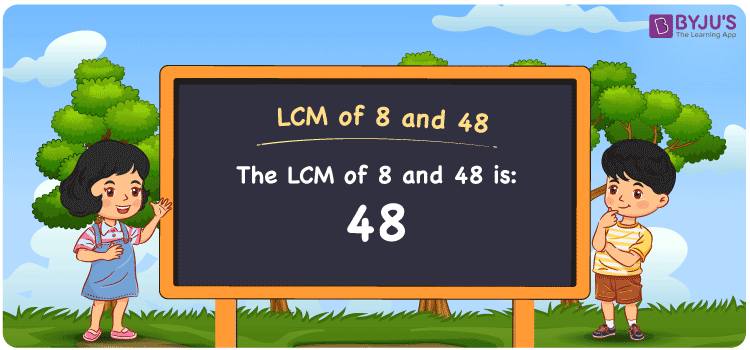# LCM of 8 and 48

LCM of 8 and 48 is 48. The smallest number among all common multiples of 8 and 48 is the LCM of 8 and 48. (8, 16, 24, 32, 40, 48, 56, etc.) and (48, 96, 144, 192, 240, 288, etc.) are the first few multiples of 8 and 48, respectively. To find the LCM of 8 and 48, there are three main methods: prime factorization, listing multiples, and division. The LCM of any two integers in mathematics is the value that is evenly divisible by the two values.

Also read: Least common multiple

## What is LCM of 8 and 48?

The answer to this question is 48. The LCM of 8 and 48 using various methods is shown in this article for your reference. The LCM of two non-zero integers, 8 and 48, is the smallest positive integer 48 which is divisible by both 8 and 48 with no remainder.## How to Find LCM of 8 and 48?

LCM of 8 and 48 can be found using three methods:

• Prime Factorisation
• Division method
• Listing the multiples

### LCM of 8 and 48 Using Prime Factorisation Method

The prime factorisation of 8 and 48, respectively, is given by:

8 = (2 × 2 × 2) = 23 and

48 = (2 × 2 × 2 × 2 × 3) = 24 × 31

LCM (8, 48) = 48

### LCM of 8 and 48 Using Division Method

We’ll divide the numbers (8, 48) by their prime factors to get the LCM of 8 and 48 using the division method (preferably common). The LCM of 8 and 48 is calculated by multiplying these divisors.

 2 8 48 2 4 24 2 2 12 2 1 6 3 1 3 x 1 1

No further division can be done.

Hence, LCM (8, 48) = 48

### LCM of 8 and 48 Using Listing the Multiples

To calculate the LCM of 8 and 48 by listing out the common multiples, list the multiples as shown below

 Multiples of 8 Multiples of 48 8 48 16 96 24 144 32 192 40 240 48 288

The smallest common multiple of 8 and 48 is 48.

Therefore LCM (8, 48) = 48

## Video Lesson on Applications of LCM## LCM of 8 and 48 Solved Example

The GCD and LCM of two numbers are 8 and 48 respectively. If one number is 8, find the other number.

Solution:

Let the other number be p.

∵ GCD × LCM = 8 × p

⇒ p = (GCD × LCM)/8

⇒ p = (8 × 48)/8

⇒ p = 48

Therefore, the other number is 48.

## Frequently Asked Questions on LCM of 8 and 48

### What is the LCM of 8 and 48?

The LCM of 8 and 48 is 48. To find the least common multiple of 8 and 48, we need to find the multiples of 8 and 48 (multiples of 8 = 8, 16, 24, 32 . . . . 48; multiples of 48 = 48, 96, 144, 192) and choose the smallest multiple that is exactly divisible by 8 and 48, i.e., 48.

### List the methods used to find the LCM of 8 and 48.

The methods used to find the LCM of 8 and 48 are Prime Factorization Method, Division Method and Listing multiples.

### What is the Least Perfect Square Divisible by 8 and 48?

The least number divisible by 8 and 48 = LCM(8, 48)
LCM of 8 and 48 = 2 × 2 × 2 × 2 × 3 [Incomplete pair(s): 3] ⇒ Least perfect square divisible by each 8 and 48 = LCM(8, 48) × 3 = 144 [Square root of 144 = √144 = ±12] Therefore, 144 is the required number.

### If the LCM of 48 and 8 is 48, Find its GCF.

LCM(48, 8) × GCF(48, 8) = 48 × 8
Since the LCM of 48 and 8 = 48
⇒ 48 × GCF(48, 8) = 384
Therefore, the GCF (greatest common factor) = 384/48 = 8.

### How to Find the LCM of 8 and 48 by Prime Factorization?

To find the LCM of 8 and 48 using prime factorization, we will find the prime factors, (8 = 2 × 2 × 2) and (48 = 2 × 2 × 2 × 2 × 3). LCM of 8 and 48 is the product of prime factors raised to their respective highest exponent among the numbers 8 and 48.
⇒ LCM of 8, 48 = 24 × 31 = 48.